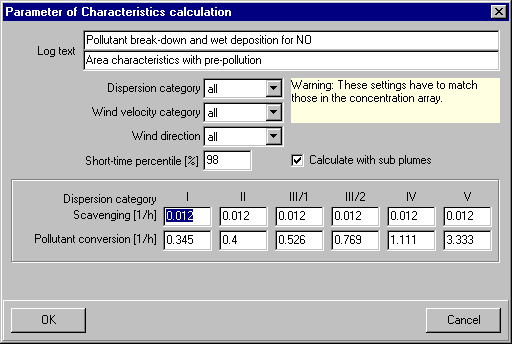# P&K 3782

## Parameter of the characteristic calculation

Product has been archived / Produkt wurde archiviert### Log text

Describing text, which will appear in the log of the characteristic calculation.

### Calculate with sub plumes

The characteristic calculation can be done without the subdivision of the wind direction 10°-sectors in 2°-sectors.

### Calculation for dispersion category

The characteristics calculation can be limited to one dispersion category. The selected categories must have been calculated in the concentration array.

### Calculation for wind velocity

The characteristic calculation can be limited to one wind velocity category. The selected categories must have been calculated in the concentration array.

### Calculation of wind direction

The characteristic calculation can be limited to one wind direction class

### Short time percentile

For the calculation of the short time percentile a value deviating from the usual 98 % can be set.

### Scavenging

For each dispersion category the definition of a scavenging coefficient is possible.

### Pollutant conversion

For each dispersion category the definition of a reaction constant (the reciprocal value of the "life time") for the break down of a pollutant is possible.

### Factor file {this only exists in a special option}

Set the full name of a file with amplifying factors.

The file must have the following line structure:

 1. line Number of operation conditions (A_Bt) 2. line Number of wind directions list (A_Wr) 3. line Number of wind velocity categories (A_Wg) 4. line Number of steps (A_St) A_Wr lines with multiple wind direction intervals at a time (in Decagrad)
 A_Bt sections A_Wr blocks A_Wg lines
with A_St amplifying factors.

All entries are unformatted, must be separated from each other with at least one blank, and must use the dot as the decimal character.
Blank lines will be ignored.